# INTELLIGENT POWERTRAIN DESIGN The BOND GRAPH Methodology for

• Slides: 44
Download presentation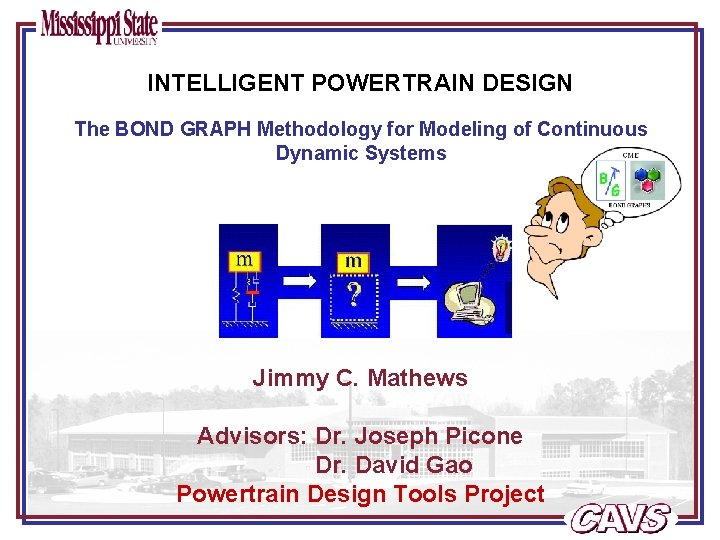INTELLIGENT POWERTRAIN DESIGN The BOND GRAPH Methodology for Modeling of Continuous Dynamic Systems Jimmy C. Mathews Advisors: Dr. Joseph Picone Dr. David Gao Powertrain Design Tools ProjectOutline • Dynamic Systems and Modeling • Bond Graph Modeling Concepts • Sample Applications of Bond Graph Modeling • The Generic Modeling Environment (GME) and Bond Graph Modeling • Future Concepts Intelligent Powertrain Design Page 1 of 43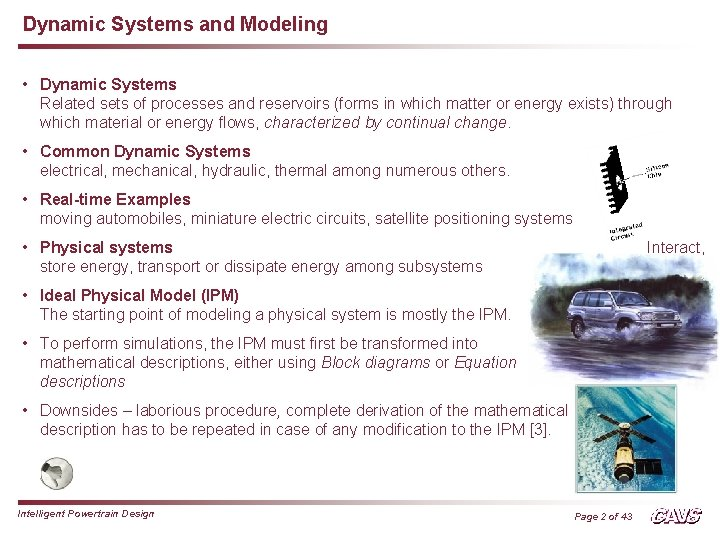Dynamic Systems and Modeling • Dynamic Systems Related sets of processes and reservoirs (forms in which matter or energy exists) through which material or energy flows, characterized by continual change. • Common Dynamic Systems electrical, mechanical, hydraulic, thermal among numerous others. • Real-time Examples moving automobiles, miniature electric circuits, satellite positioning systems • Physical systems store energy, transport or dissipate energy among subsystems Interact, • Ideal Physical Model (IPM) The starting point of modeling a physical system is mostly the IPM. • To perform simulations, the IPM must first be transformed into mathematical descriptions, either using Block diagrams or Equation descriptions • Downsides – laborious procedure, complete derivation of the mathematical description has to be repeated in case of any modification to the IPM . Intelligent Powertrain Design Page 2 of 43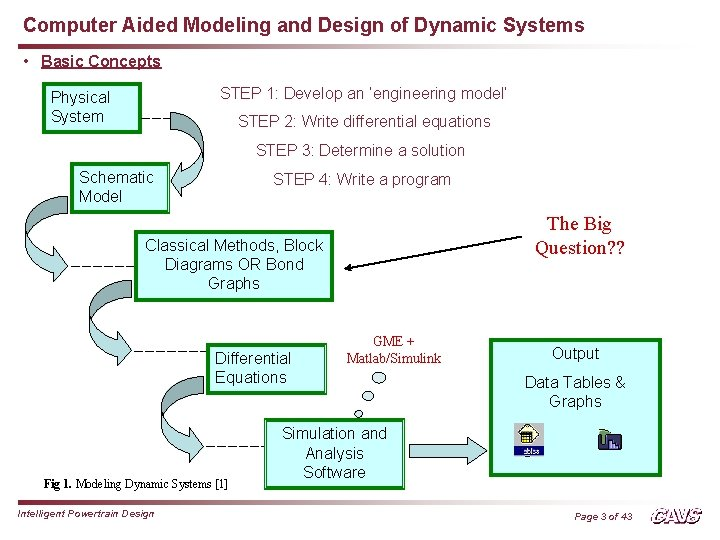Computer Aided Modeling and Design of Dynamic Systems • Basic Concepts STEP 1: Develop an ‘engineering model’ Physical System STEP 2: Write differential equations STEP 3: Determine a solution Schematic Model STEP 4: Write a program The Big Question? ? Classical Methods, Block Diagrams OR Bond Graphs Differential Equations Fig 1. Modeling Dynamic Systems  Intelligent Powertrain Design GME + Matlab/Simulink Output Data Tables & Graphs Simulation and Analysis Software Page 3 of 43Bond Graphs vs. Block Diagrams [5, 8] • Block Diagrams Early attempt to deal with heterogeneity, closely related to the emergence of automatic control, nice example of information hiding, very successful and good environments like Simulink, Easy V, and Vis. Sim available presently. • Familiar and versatile graphical notation to represent Signal Flow. • Downsides i. Do not provide a suitable notation for depicting physical system models because not all block diagrams represent physical processes. ii. Energetic Coupling between elements/systems - - - energy exchange implies interaction, i. e. a bilateral, two-way influence of each system on the other. Block diagrams fundamentally depict a unilateral influence of one system on another. Hence, to describe energetic interaction of two systems/elements in terms of signal flow, the output of one should be the input of another and vice versa. iii. When two systems interact energetically, we must have the block representation as in figure 2 (or its converse). In contrast, the block diagrams shown below might represent Fig 2. Block Diagram of Energetic possible operations on signals or information, but neither Interaction  represents any possible energetic interaction. Intelligent Powertrain Design Page 4 of 43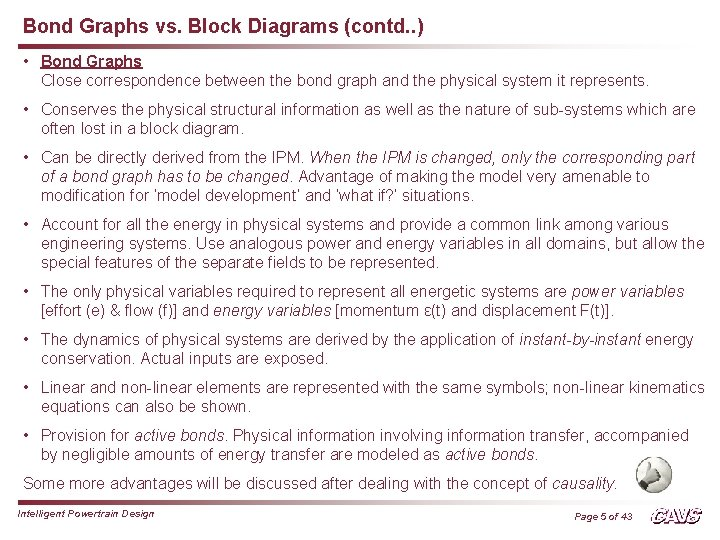Bond Graphs vs. Block Diagrams (contd. . ) • Bond Graphs Close correspondence between the bond graph and the physical system it represents. • Conserves the physical structural information as well as the nature of sub-systems which are often lost in a block diagram. • Can be directly derived from the IPM. When the IPM is changed, only the corresponding part of a bond graph has to be changed. Advantage of making the model very amenable to modification for ‘model development’ and ‘what if? ’ situations. • Account for all the energy in physical systems and provide a common link among various engineering systems. Use analogous power and energy variables in all domains, but allow the special features of the separate fields to be represented. • The only physical variables required to represent all energetic systems are power variables [effort (e) & flow (f)] and energy variables [momentum ε(t) and displacement F(t)]. • The dynamics of physical systems are derived by the application of instant-by-instant energy conservation. Actual inputs are exposed. • Linear and non-linear elements are represented with the same symbols; non-linear kinematics equations can also be shown. • Provision for active bonds. Physical information involving information transfer, accompanied by negligible amounts of energy transfer are modeled as active bonds. Some more advantages will be discussed after dealing with the concept of causality. Intelligent Powertrain Design Page 5 of 43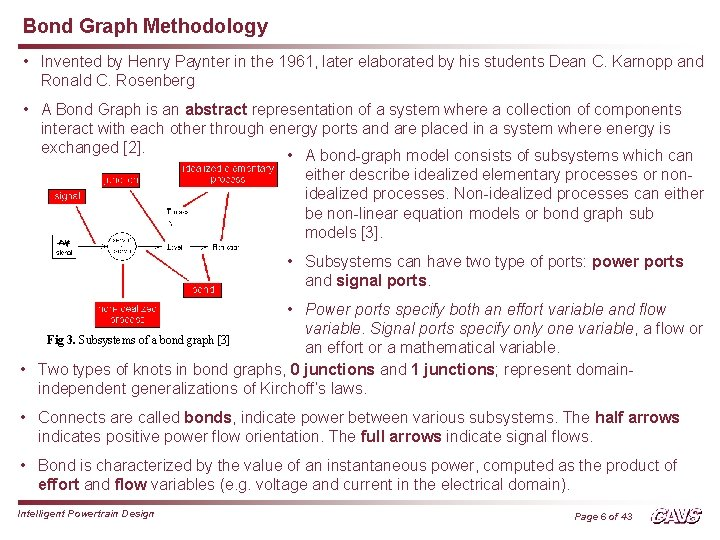Bond Graph Methodology • Invented by Henry Paynter in the 1961, later elaborated by his students Dean C. Karnopp and Ronald C. Rosenberg • A Bond Graph is an abstract representation of a system where a collection of components interact with each other through energy ports and are placed in a system where energy is exchanged . • A bond-graph model consists of subsystems which can either describe idealized elementary processes or nonidealized processes. Non-idealized processes can either be non-linear equation models or bond graph sub models . • Subsystems can have two type of ports: power ports and signal ports. • Power ports specify both an effort variable and flow variable. Signal ports specify only one variable, a flow or Fig 3. Subsystems of a bond graph  an effort or a mathematical variable. • Two types of knots in bond graphs, 0 junctions and 1 junctions; represent domainindependent generalizations of Kirchoff’s laws. • Connects are called bonds, indicate power between various subsystems. The half arrows indicates positive power flow orientation. The full arrows indicate signal flows. • Bond is characterized by the value of an instantaneous power, computed as the product of effort and flow variables (e. g. voltage and current in the electrical domain). Intelligent Powertrain Design Page 6 of 43The Bond Graph Modeling Formalism • Bond Graph’s Reach? Mechanical Rotation Hydraulic/Pneumatic Mechanical Translation Electrical Magnetic Intelligent Powertrain Design Thermal Chemical/Process Engineering Fig 4. Multi-Energy Systems Modeling using Bond Graphs Page 7 of 43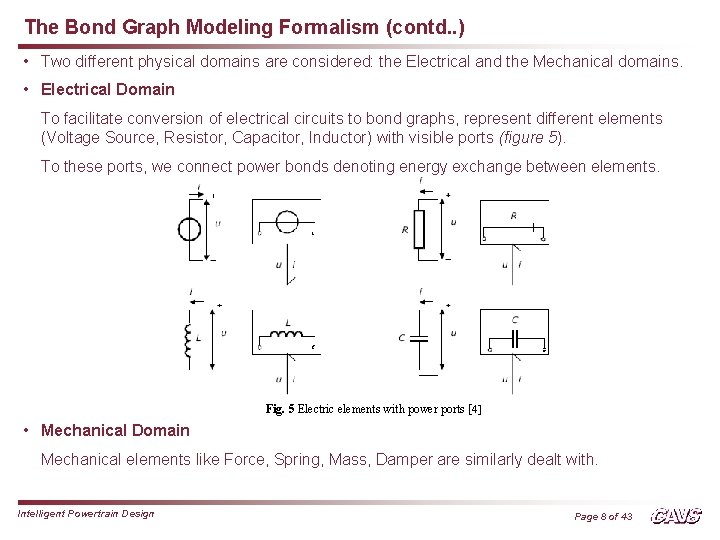The Bond Graph Modeling Formalism (contd. . ) • Two different physical domains are considered: the Electrical and the Mechanical domains. • Electrical Domain To facilitate conversion of electrical circuits to bond graphs, represent different elements (Voltage Source, Resistor, Capacitor, Inductor) with visible ports (figure 5). To these ports, we connect power bonds denoting energy exchange between elements. Fig. 5 Electric elements with power ports [ 4] • Mechanical Domain Mechanical elements like Force, Spring, Mass, Damper are similarly dealt with. Intelligent Powertrain Design Page 8 of 43The Bond Graph Modeling Formalism (contd. . ) The R – L - C circuit The power being exchanged by a port with the rest of the system is a product of the voltage and the current: P=u*i The equations for the resistor, capacitor and inductor are: u_R = i * R u_C = 1/C * (∫idt) u_L = L * (di/dt); or i = 1/L * (∫u_L dt) 1 Fig 6. The RLC Circuit  Intelligent Powertrain Design Page 9 of 43The Bond Graph Modeling Formalism (contd. . ) The Spring-Mass-Damper System Port variables on the bond graph elements are force on the element port and velocity of the element port. P=F*v The equations for the damper (damping coefficient, α), spring (coefficient, KS) and mass are: F_d = α * v F_s = KS * (∫v dt) = 1/CS * (∫ vdt) F_m = m * (dv/dt); or v = 1/m * (∫F_m dt); Also, F_a = force Fig 7. The Spring Mass Damper System  Intelligent Powertrain Design Page 10 of 43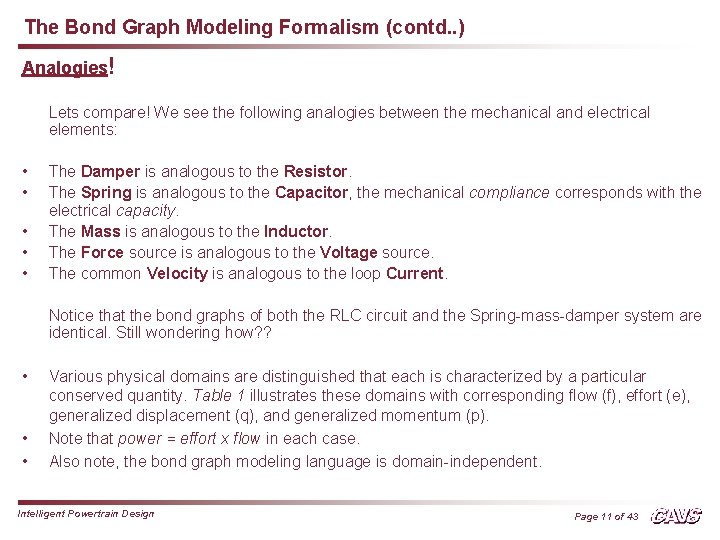The Bond Graph Modeling Formalism (contd. . ) Analogies! Lets compare! We see the following analogies between the mechanical and electrical elements: • • • The Damper is analogous to the Resistor. The Spring is analogous to the Capacitor, the mechanical compliance corresponds with the electrical capacity. The Mass is analogous to the Inductor. The Force source is analogous to the Voltage source. The common Velocity is analogous to the loop Current. Notice that the bond graphs of both the RLC circuit and the Spring-mass-damper system are identical. Still wondering how? ? • • • Various physical domains are distinguished that each is characterized by a particular conserved quantity. Table 1 illustrates these domains with corresponding flow (f), effort (e), generalized displacement (q), and generalized momentum (p). Note that power = effort x flow in each case. Also note, the bond graph modeling language is domain-independent. Intelligent Powertrain Design Page 11 of 43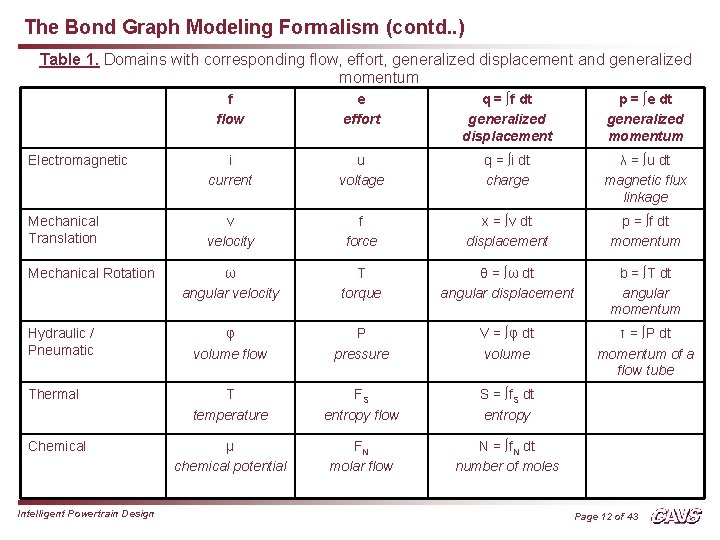The Bond Graph Modeling Formalism (contd. . ) Table 1. Domains with corresponding flow, effort, generalized displacement and generalized momentum f flow e effort q = ∫f dt generalized displacement p = ∫e dt generalized momentum Electromagnetic i current u voltage q = ∫i dt charge λ = ∫u dt magnetic flux linkage Mechanical Translation v velocity f force x = ∫v dt displacement p = ∫f dt momentum ω angular velocity T torque θ = ∫ω dt angular displacement b = ∫T dt angular momentum Hydraulic / Pneumatic φ volume flow P pressure V = ∫φ dt volume τ = ∫P dt momentum of a flow tube Thermal T temperature FS entropy flow S = ∫f. S dt entropy Chemical μ chemical potential FN molar flow N = ∫f. N dt number of moles Mechanical Rotation Intelligent Powertrain Design Page 12 of 43The Bond Graph Modeling Formalism (contd. . ) • Foundations of Bond Graphs Based on the assumptions that satisfy basic principles of physics; a. Law of Energy Conservation is applicable b. Positive Entropy production Power Continuity • Closer look at Bonds and Ports Fig. 8 Energy flow between two sub models represented by a bond  c. Power port or port: The contact point of a sub model where an ideal connection will be connected. Power bond or bond: The connection between two sub models; drawn by a single line (Fig. 8) Bond denotes ideal energy flow between two sub models; the energy entering the bond on one side immediately leaves the bond at the other side (power continuity). Energy flow along the bond has the physical dimension of power, being the product of two variables; called power-conjugated variables. A e f B (directed bond from A to B) Intelligent Powertrain Design Page 13 of 43The Bond Graph Modeling Formalism (contd. . ) • Two views of Interpretation of Power Bond 1. As an interaction of energy; connected subsystems for a load to each other by their energy exchange; embodies an exchange of a physical quantity. 2. As a bilateral signal flow; interpreted as effort and flowing in opposite direction, thus determining the computational direction of the bond variables; w. r. t. one of the sub models, effort is the input and flow is the output and vice versa for the other sub model. • Determining the direction of Effort and Flow During modeling it need not be decided what the computational direction of the bond variables is, however it is necessary to derive the mathematical model (set of differential equations) from the graph. Process of determining the computational direction of the bond variables is called causal analysis; indicated in the graph by the so-called causal stroke, (indicating the direction of the effort), called the causality of the bond (figure 9). Fig. 9 Why is the Power direction not shown?  Intelligent Powertrain Design Page 14 of 43The Bond Graph Modeling Formalism (contd. . ) • Bond Graph Elements Bond graph elements are drawn as letter combinations (mnemonic codes) indicating the type of element. The bond graph elements are the following: C storage element for a q-type variable, e. g. capacitor (stores charge), spring (stores displacement) L storage element for a p-type variable, e. g. inductor (stores flux linkage), mass (stores momentum) R resistor dissipating free energy, e. g. electric resistor, mechanical friction Se, Sf sources, e. g. electric mains (voltage source), gravity (force source), pump (flow source) TF transformer, e. g. an electric transformer, toothed wheels, lever GY gyrator, e. g. electromotor, centrifugal pump 0, 1 0 and 1–junctions, for ideal connecting two or more sub models Intelligent Powertrain Design Page 15 of 43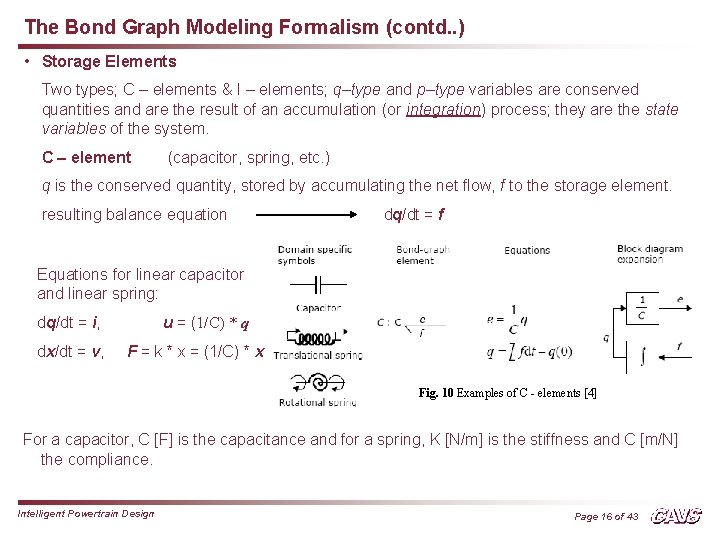The Bond Graph Modeling Formalism (contd. . ) • Storage Elements Two types; C – elements & I – elements; q–type and p–type variables are conserved quantities and are the result of an accumulation (or integration) process; they are the state variables of the system. C – element (capacitor, spring, etc. ) q is the conserved quantity, stored by accumulating the net flow, f to the storage element. resulting balance equation dq/dt = f Equations for linear capacitor and linear spring: dq/dt = i, dx/dt = v, u = (1/C) * q F = k * x = (1/C) * x Fig. 10 Examples of C - elements  For a capacitor, C [F] is the capacitance and for a spring, K [N/m] is the stiffness and C [m/N] the compliance. Intelligent Powertrain Design Page 16 of 43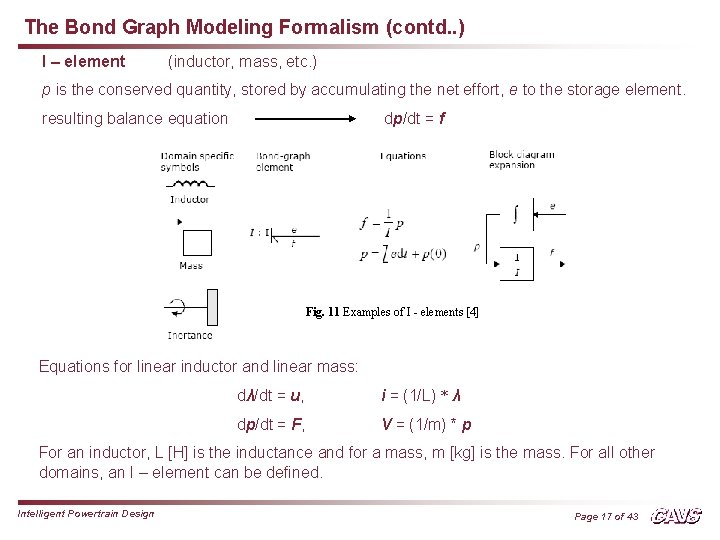The Bond Graph Modeling Formalism (contd. . ) I – element (inductor, mass, etc. ) p is the conserved quantity, stored by accumulating the net effort, e to the storage element. resulting balance equation dp/dt = f Fig. 11 Examples of I - elements  Equations for linear inductor and linear mass: dλ/dt = u, i = (1/L) * λ dp/dt = F, V = (1/m) * p For an inductor, L [H] is the inductance and for a mass, m [kg] is the mass. For all other domains, an I – element can be defined. Intelligent Powertrain Design Page 17 of 43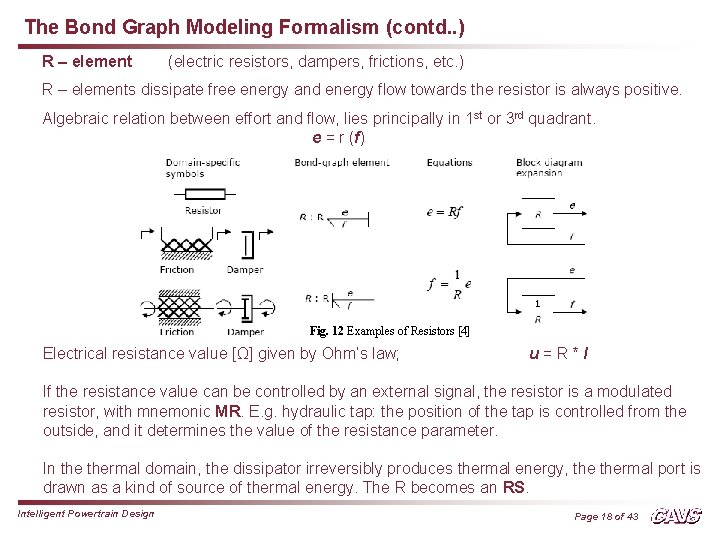The Bond Graph Modeling Formalism (contd. . ) R – element (electric resistors, dampers, frictions, etc. ) R – elements dissipate free energy and energy flow towards the resistor is always positive. Algebraic relation between effort and flow, lies principally in 1 st or 3 rd quadrant. e = r (f) Fig. 12 Examples of Resistors  Electrical resistance value [Ω] given by Ohm’s law; u=R*I If the resistance value can be controlled by an external signal, the resistor is a modulated resistor, with mnemonic MR. E. g. hydraulic tap: the position of the tap is controlled from the outside, and it determines the value of the resistance parameter. In thermal domain, the dissipator irreversibly produces thermal energy, thermal port is drawn as a kind of source of thermal energy. The R becomes an RS. Intelligent Powertrain Design Page 18 of 43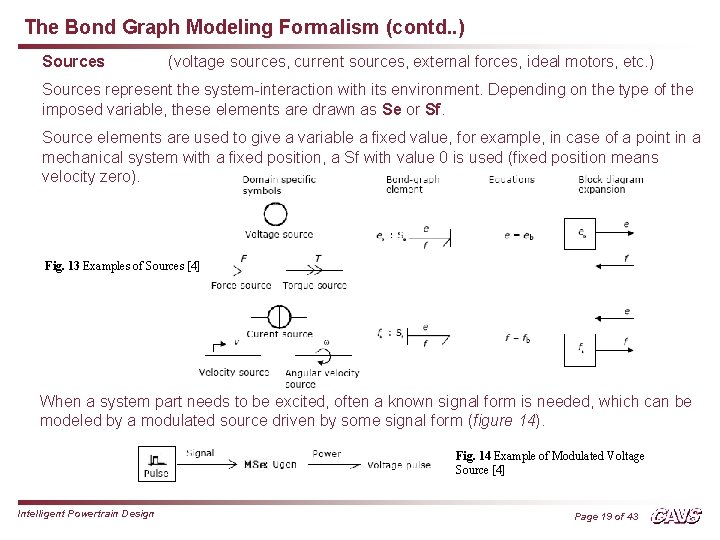The Bond Graph Modeling Formalism (contd. . ) Sources (voltage sources, current sources, external forces, ideal motors, etc. ) Sources represent the system-interaction with its environment. Depending on the type of the imposed variable, these elements are drawn as Se or Sf. Source elements are used to give a variable a fixed value, for example, in case of a point in a mechanical system with a fixed position, a Sf with value 0 is used (fixed position means velocity zero). Fig. 13 Examples of Sources  When a system part needs to be excited, often a known signal form is needed, which can be modeled by a modulated source driven by some signal form (figure 14). Fig. 14 Example of Modulated Voltage Source  Intelligent Powertrain Design Page 19 of 43The Bond Graph Modeling Formalism (contd. . ) Transformers (toothed wheel, electromotor, etc. ) An ideal transformer is represented by TF and is power continuous (i. e. no power is stored or dissipated). The transformations can be within the same domain (toothed wheel, lever) or between different domains (electromotor, winch). e 1 = n * e 2 & f 2 = n * f 1 Efforts are transduced to efforts and flows to flows; n is the transformer ratio. Only one dimensionless parameter n is required to describe effort transduction and flow transduction. n is a defined as follows: e 1 and f 1 belong to the bond pointing towards TF. Fig. 15 Examples of Transformers  If n is not constant, it becomes an input signal to the modulated transformer, MTF. Intelligent Powertrain Design Page 20 of 43The Bond Graph Modeling Formalism (contd. . ) Gyrators (electromotor, pump, turbine) An ideal gyrator is represented by GY and is power continuous (i. e. no power is stored or dissipated). Real-life realizations of gyrators are mostly transducers representing a domaintransformation. e 1 = r * f 2 & e 2 = r * f 1 r is the gyrator ratio and is the only parameter required to describe both equations. R has a physical dimension (same as R-element), since r is the relation between effort and flow. Fig. 16 Examples of Gyrators  Gyrator is defined by one bond pointing towards and other bond pointing away. If r is not constant, the gyrator is a modulated gyrator, a MGY. Intelligent Powertrain Design Page 21 of 43The Bond Graph Modeling Formalism (contd. . ) Junctions couple two or more elements in a power continuous way; there is no storage or dissipation at a junction. 0 – junction Represents a node at which all efforts of the connecting bonds are equal. E. g. a parallel connection in an electrical circuit. The sum of flows of the connecting bonds is zero, considering the sign. The power direction determines the sign of flows: all inward pointing bonds get a plus and all outward pointing bonds get a minus. 0 – junction can be interpreted as the generalized Kirchoff’s Current Law. Additionally, equality of efforts (like electrical voltage) at a parallel connection. Fig. 17 Example of a 0 Junction  Intelligent Powertrain Design Page 22 of 43The Bond Graph Modeling Formalism (contd. . ) 1 – junction Is the dual form of the 0 -junction (roles of effort and flow are exchanged). Represents a node at which all flows of the connecting bonds are equal. E. g. a series connection in an electrical circuit. The efforts of the connecting bonds sum to zero. Again, the power direction determines the sign of flows: all inward pointing bonds get a plus and all outward pointing bonds get a minus. 1 - junction can be interpreted as the generalized Kirchoff’s Voltage Law. In the mechanical domain, 1 -junction represents a force-balance, and is a generalization of Newton’ third law. Additionally, equality of flows (like electrical current) through a series connection. Fig. 18 Example of a 1 -Junction  Intelligent Powertrain Design Page 23 of 43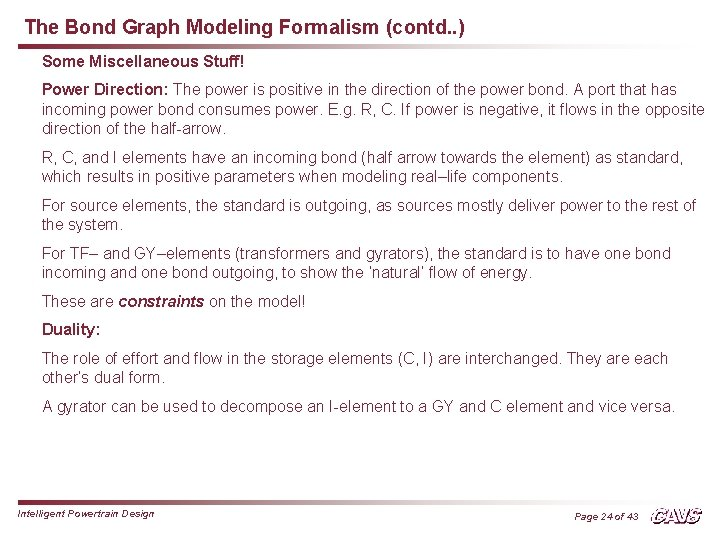The Bond Graph Modeling Formalism (contd. . ) Some Miscellaneous Stuff! Power Direction: The power is positive in the direction of the power bond. A port that has incoming power bond consumes power. E. g. R, C. If power is negative, it flows in the opposite direction of the half-arrow. R, C, and I elements have an incoming bond (half arrow towards the element) as standard, which results in positive parameters when modeling real–life components. For source elements, the standard is outgoing, as sources mostly deliver power to the rest of the system. For TF– and GY–elements (transformers and gyrators), the standard is to have one bond incoming and one bond outgoing, to show the ‘natural’ flow of energy. These are constraints on the model! Duality: The role of effort and flow in the storage elements (C, I) are interchanged. They are each other’s dual form. A gyrator can be used to decompose an I-element to a GY and C element and vice versa. Intelligent Powertrain Design Page 24 of 43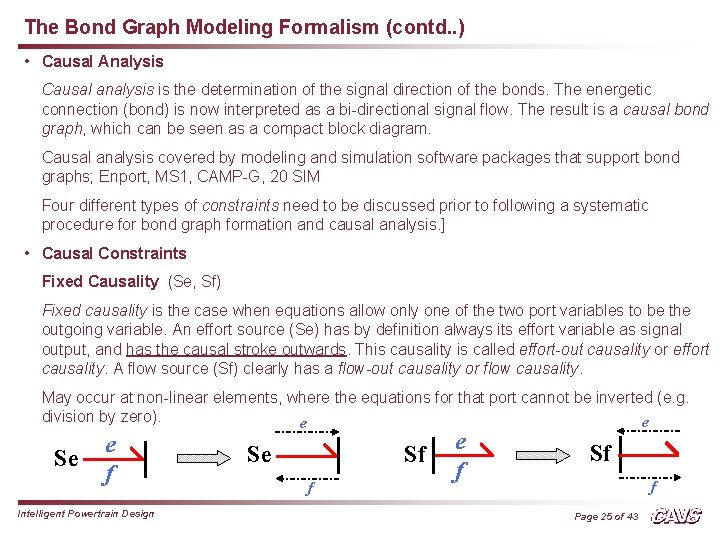The Bond Graph Modeling Formalism (contd. . ) • Causal Analysis Causal analysis is the determination of the signal direction of the bonds. The energetic connection (bond) is now interpreted as a bi-directional signal flow. The result is a causal bond graph, which can be seen as a compact block diagram. Causal analysis covered by modeling and simulation software packages that support bond graphs; Enport, MS 1, CAMP-G, 20 SIM Four different types of constraints need to be discussed prior to following a systematic procedure for bond graph formation and causal analysis. ] • Causal Constraints Fixed Causality (Se, Sf) Fixed causality is the case when equations allow only one of the two port variables to be the outgoing variable. An effort source (Se) has by definition always its effort variable as signal output, and has the causal stroke outwards. This causality is called effort-out causality or effort causality. A flow source (Sf) clearly has a flow-out causality or flow causality. May occur at non-linear elements, where the equations for that port cannot be inverted (e. g. division by zero). e e Se e f Intelligent Powertrain Design Sf Se f Sf f Page 25 of 43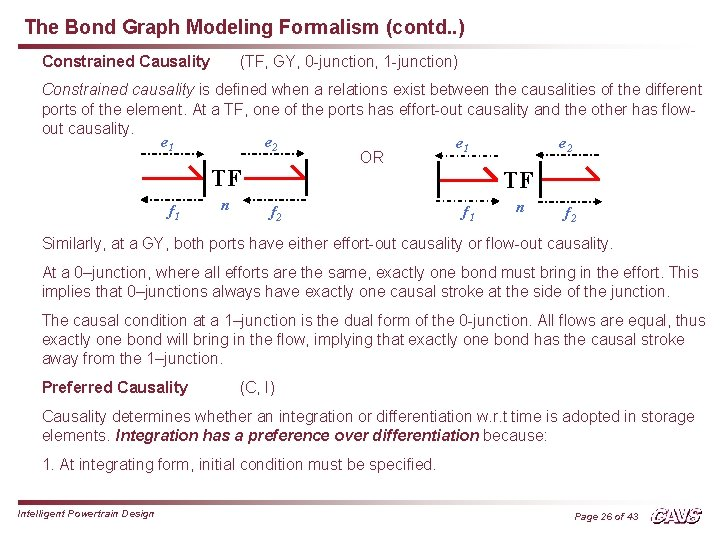The Bond Graph Modeling Formalism (contd. . ) Constrained Causality (TF, GY, 0 -junction, 1 -junction) Constrained causality is defined when a relations exist between the causalities of the different ports of the element. At a TF, one of the ports has effort-out causality and the other has flowout causality. e 2 e 1 OR TF f 1 n TF f 2 f 1 n f 2 Similarly, at a GY, both ports have either effort-out causality or flow-out causality. At a 0–junction, where all efforts are the same, exactly one bond must bring in the effort. This implies that 0–junctions always have exactly one causal stroke at the side of the junction. The causal condition at a 1–junction is the dual form of the 0 -junction. All flows are equal, thus exactly one bond will bring in the flow, implying that exactly one bond has the causal stroke away from the 1–junction. Preferred Causality (C, I) Causality determines whether an integration or differentiation w. r. t time is adopted in storage elements. Integration has a preference over differentiation because: 1. At integrating form, initial condition must be specified. Intelligent Powertrain Design Page 26 of 43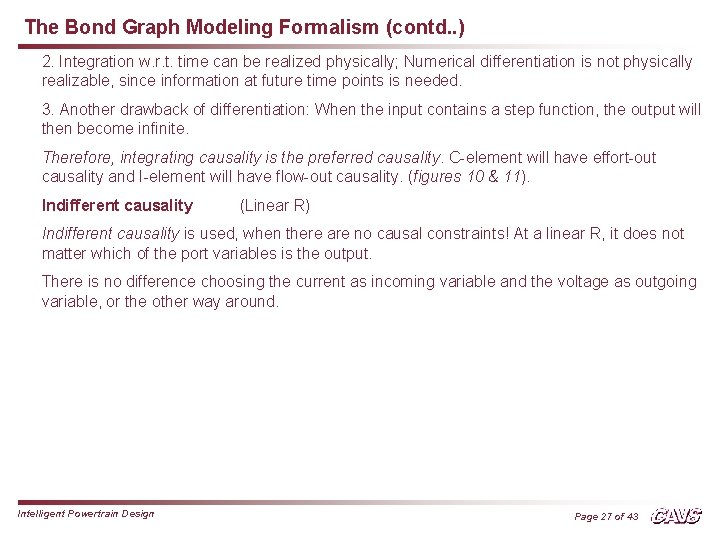The Bond Graph Modeling Formalism (contd. . ) 2. Integration w. r. t. time can be realized physically; Numerical differentiation is not physically realizable, since information at future time points is needed. 3. Another drawback of differentiation: When the input contains a step function, the output will then become infinite. Therefore, integrating causality is the preferred causality. C-element will have effort-out causality and I-element will have flow-out causality. (figures 10 & 11). Indifferent causality (Linear R) Indifferent causality is used, when there are no causal constraints! At a linear R, it does not matter which of the port variables is the output. There is no difference choosing the current as incoming variable and the voltage as outgoing variable, or the other way around. Intelligent Powertrain Design Page 27 of 43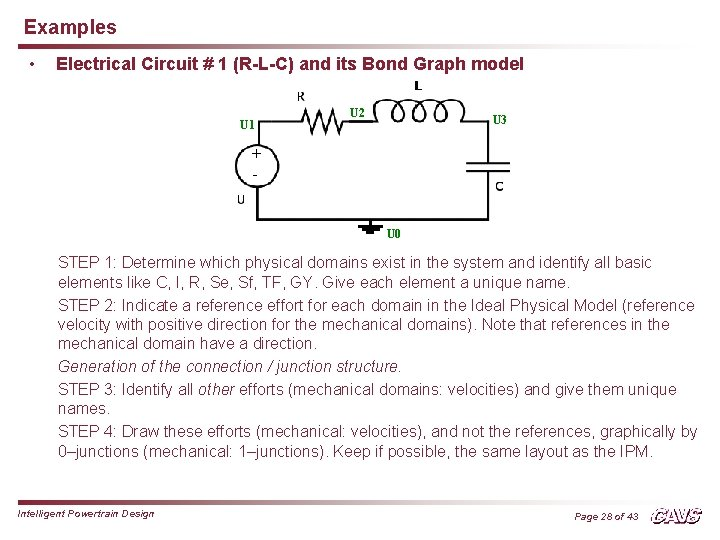Examples • Electrical Circuit # 1 (R-L-C) and its Bond Graph model U 1 U 2 U 3 + - U 0 STEP 1: Determine which physical domains exist in the system and identify all basic elements like C, I, R, Se, Sf, TF, GY. Give each element a unique name. STEP 2: Indicate a reference effort for each domain in the Ideal Physical Model (reference velocity with positive direction for the mechanical domains). Note that references in the mechanical domain have a direction. Generation of the connection / junction structure. STEP 3: Identify all other efforts (mechanical domains: velocities) and give them unique names. STEP 4: Draw these efforts (mechanical: velocities), and not the references, graphically by 0–junctions (mechanical: 1–junctions). Keep if possible, the same layout as the IPM. Intelligent Powertrain Design Page 28 of 43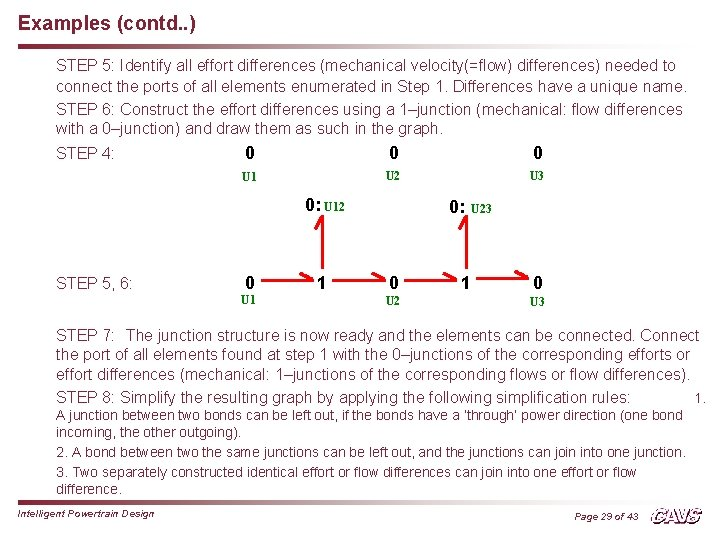Examples (contd. . ) STEP 5: Identify all effort differences (mechanical velocity(=flow) differences) needed to connect the ports of all elements enumerated in Step 1. Differences have a unique name. STEP 6: Construct the effort differences using a 1–junction (mechanical: flow differences with a 0–junction) and draw them as such in the graph. STEP 4: 0 0 0 U 1 U 2 U 3 0: U 12 STEP 5, 6: 0 U 1 1 0: 0 U 23 1 0 U 3 STEP 7: The junction structure is now ready and the elements can be connected. Connect the port of all elements found at step 1 with the 0–junctions of the corresponding efforts or effort differences (mechanical: 1–junctions of the corresponding flows or flow differences). STEP 8: Simplify the resulting graph by applying the following simplification rules: 1. A junction between two bonds can be left out, if the bonds have a ‘through’ power direction (one bond incoming, the other outgoing). 2. A bond between two the same junctions can be left out, and the junctions can join into one junction. 3. Two separately constructed identical effort or flow differences can join into one effort or flow difference. Intelligent Powertrain Design Page 29 of 43Examples (contd. . ) STEP 7: Se : U 0 R: R I: L 0: U 12 0: U 23 1 0 U 3 U 2 U 1 C: C STEP 8: R: R Se : U 1 I: L C: C Intelligent Powertrain Design Page 30 of 43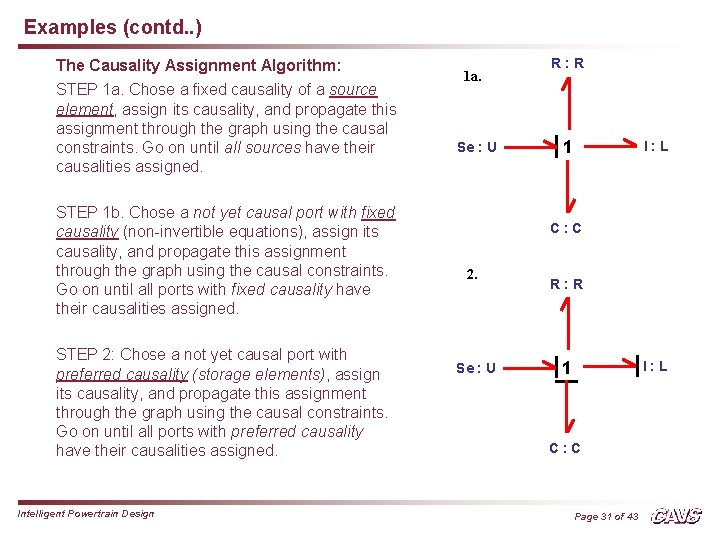Examples (contd. . ) The Causality Assignment Algorithm: STEP 1 a. Chose a fixed causality of a source element, assign its causality, and propagate this assignment through the graph using the causal constraints. Go on until all sources have their causalities assigned. STEP 1 b. Chose a not yet causal port with fixed causality (non-invertible equations), assign its causality, and propagate this assignment through the graph using the causal constraints. Go on until all ports with fixed causality have their causalities assigned. STEP 2: Chose a not yet causal port with preferred causality (storage elements), assign its causality, and propagate this assignment through the graph using the causal constraints. Go on until all ports with preferred causality have their causalities assigned. Intelligent Powertrain Design 1 a. Se : U R: R 1 I: L C: C 2. Se : U R: R 1 I: L C: C Page 31 of 43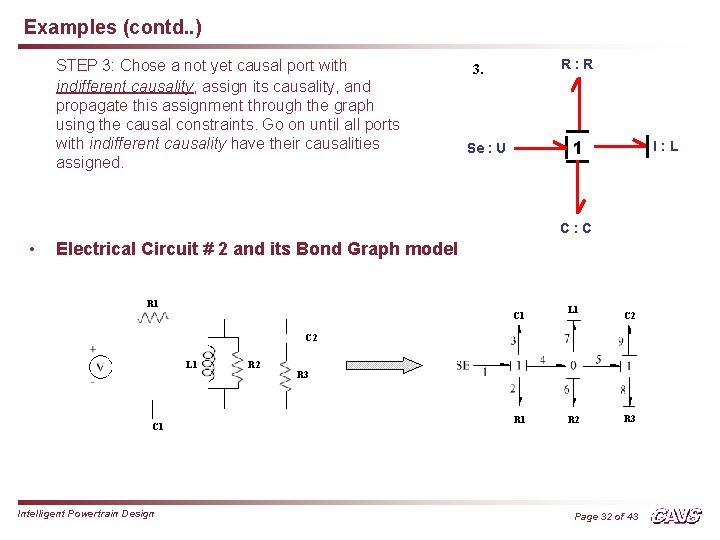Examples (contd. . ) STEP 3: Chose a not yet causal port with indifferent causality, assign its causality, and propagate this assignment through the graph using the causal constraints. Go on until all ports with indifferent causality have their causalities assigned. R: R 3. 1 Se : U I: L C: C • Electrical Circuit # 2 and its Bond Graph model R 1 C 1 L 1 C 2 L 1 C 1 Intelligent Powertrain Design R 2 R 3 R 1 R 2 R 3 Page 32 of 43Examples (contd. . ) • A DC Motor and its Bond Graph model Se 2: τ Se 1: Ua Km Intelligent Powertrain Design Page 33 of 43Generation of Equations from Bond Graphs Se : U R: R • 2 • 1 1 4 I: L 3 • • C: C Fig. 19 Bond Graph of a series RLC circuit • A causal bond graph contains all information to derive the set of state equations. Either a set of Ordinary first-order Differential Equations (ODE) or a set of Differential and Algebraic Equations (DAE). Write the set of mixed differential and algebraic equations. For a bond graph with n bonds, 2 n equations can be formed, n equations each to compute effort and flow or their derivatives. Then, the algebraic equations are eliminated, to get final equations in state-variable form. For the given RLC circuit, Se = e 1= U; e 2 = R * f 2; (de 3/dt) = (1/C) * f 3; (df 4/dt) = (1/L) * e 4; f 1 = f 4; f 2 = f 4; f 3 = f 4; e 4 = e 1 - e 2 - e 3 Hence, e 1 - e 2 - e 3 = U – (R * f 2) – e 3 = U – (R * f 4) – e 3 (df 4/dt) = (1/L) * (U – (R * f 4) – e 3) - - - - (i) Intelligent Powertrain Design Page 34 of 43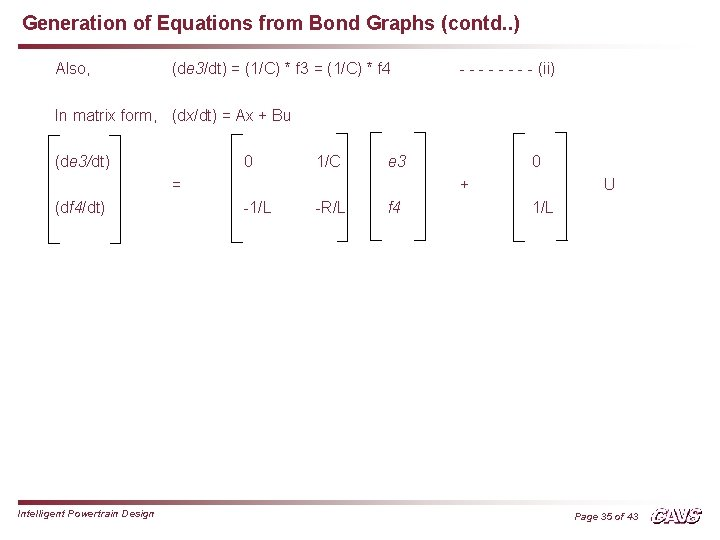Generation of Equations from Bond Graphs (contd. . ) Also, (de 3/dt) = (1/C) * f 3 = (1/C) * f 4 - - - - (ii) In matrix form, (dx/dt) = Ax + Bu (de 3/dt) 0 1/C e 3 = (df 4/dt) Intelligent Powertrain Design 0 + -1/L -R/L f 4 U 1/L Page 35 of 43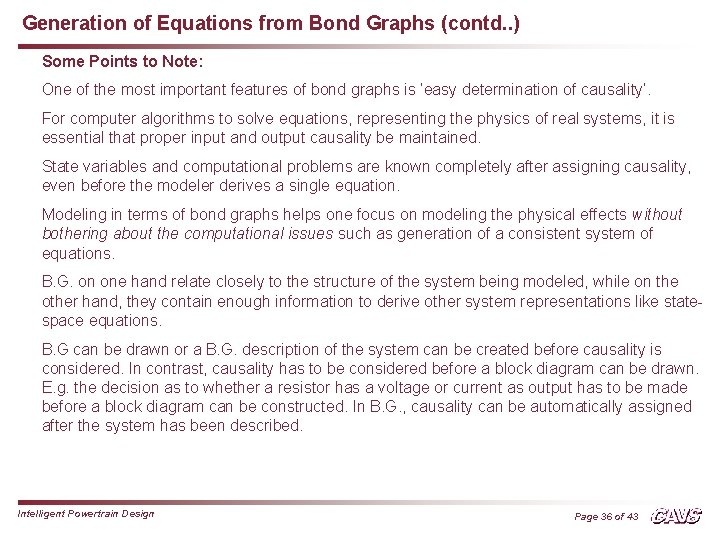Generation of Equations from Bond Graphs (contd. . ) Some Points to Note: One of the most important features of bond graphs is ‘easy determination of causality’. For computer algorithms to solve equations, representing the physics of real systems, it is essential that proper input and output causality be maintained. State variables and computational problems are known completely after assigning causality, even before the modeler derives a single equation. Modeling in terms of bond graphs helps one focus on modeling the physical effects without bothering about the computational issues such as generation of a consistent system of equations. B. G. on one hand relate closely to the structure of the system being modeled, while on the other hand, they contain enough information to derive other system representations like statespace equations. B. G can be drawn or a B. G. description of the system can be created before causality is considered. In contrast, causality has to be considered before a block diagram can be drawn. E. g. the decision as to whether a resistor has a voltage or current as output has to be made before a block diagram can be constructed. In B. G. , causality can be automatically assigned after the system has been described. Intelligent Powertrain Design Page 36 of 43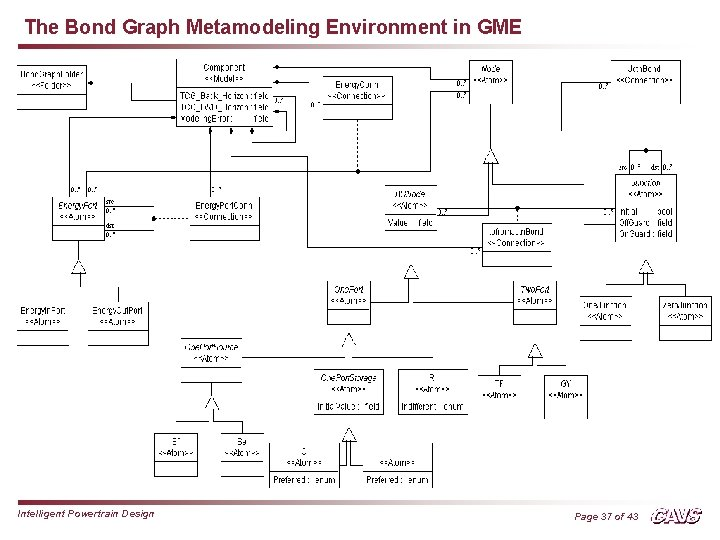The Bond Graph Metamodeling Environment in GME Intelligent Powertrain Design Page 37 of 43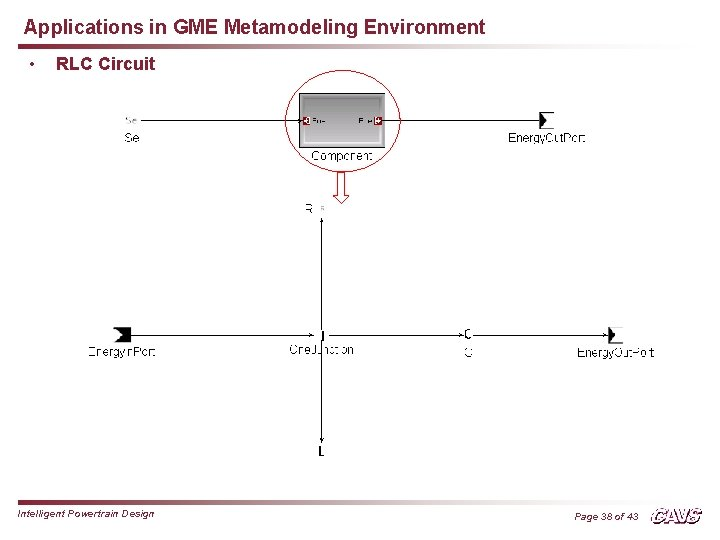Applications in GME Metamodeling Environment • RLC Circuit Intelligent Powertrain Design Page 38 of 43Applications in GME Metamodeling Environment (contd. . ) DC Motor model Intelligent Powertrain Design Page 39 of 43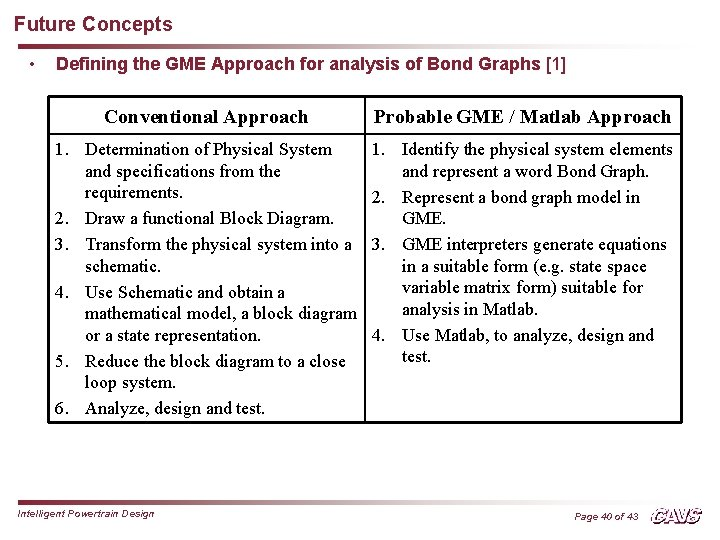Future Concepts • Defining the GME Approach for analysis of Bond Graphs  Conventional Approach Probable GME / Matlab Approach 1. Determination of Physical System and specifications from the requirements. 2. Draw a functional Block Diagram. 3. Transform the physical system into a schematic. 4. Use Schematic and obtain a mathematical model, a block diagram or a state representation. 5. Reduce the block diagram to a close loop system. 6. Analyze, design and test. 1. Identify the physical system elements and represent a word Bond Graph. 2. Represent a bond graph model in GME. 3. GME interpreters generate equations in a suitable form (e. g. state space variable matrix form) suitable for analysis in Matlab. 4. Use Matlab, to analyze, design and test. Intelligent Powertrain Design Page 40 of 43Future Concepts (contd. . ) • Creating Bond Graph Interpreters in GME ? ? Fig. The Simulation Generation Process  Intelligent Powertrain Design Page 41 of 43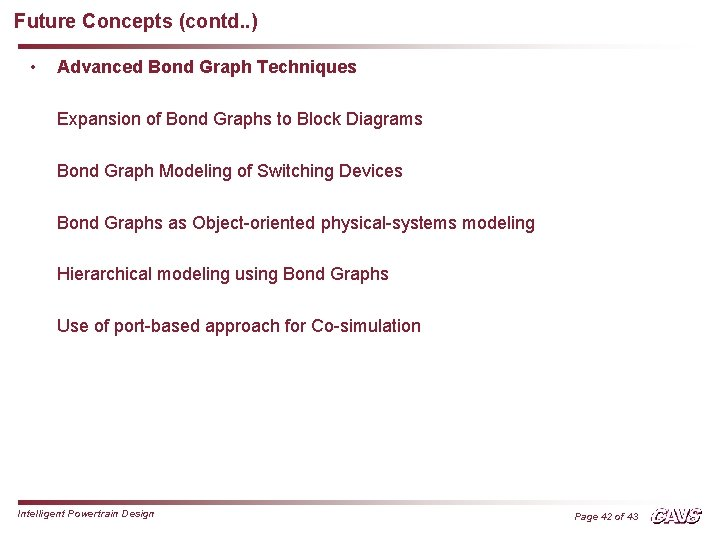Future Concepts (contd. . ) • Advanced Bond Graph Techniques Expansion of Bond Graphs to Block Diagrams Bond Graph Modeling of Switching Devices Bond Graphs as Object-oriented physical-systems modeling Hierarchical modeling using Bond Graphs Use of port-based approach for Co-simulation Intelligent Powertrain Design Page 42 of 43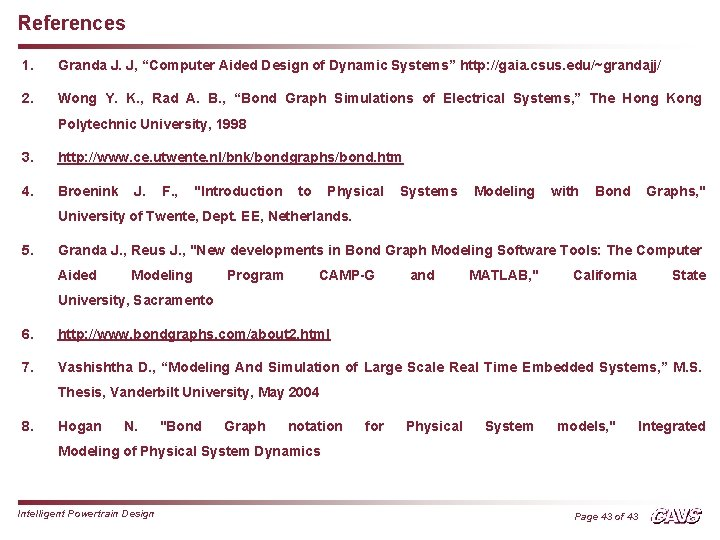References 1. Granda J. J, “Computer Aided Design of Dynamic Systems” http: //gaia. csus. edu/~grandajj/ 2. Wong Y. K. , Rad A. B. , “Bond Graph Simulations of Electrical Systems, ” The Hong Kong Polytechnic University, 1998 3. http: //www. ce. utwente. nl/bnk/bondgraphs/bond. htm 4. Broenink J. F. , "Introduction to Physical Systems Modeling with Bond Graphs, " University of Twente, Dept. EE, Netherlands. 5. Granda J. , Reus J. , "New developments in Bond Graph Modeling Software Tools: The Computer Aided Modeling Program CAMP-G and MATLAB, " California State University, Sacramento 6. http: //www. bondgraphs. com/about 2. html 7. Vashishtha D. , “Modeling And Simulation of Large Scale Real Time Embedded Systems, ” M. S. Thesis, Vanderbilt University, May 2004 8. Hogan N. "Bond Graph notation for Physical System models, " Modeling of Physical System Dynamics Intelligent Powertrain Design Page 43 of 43 Integrated